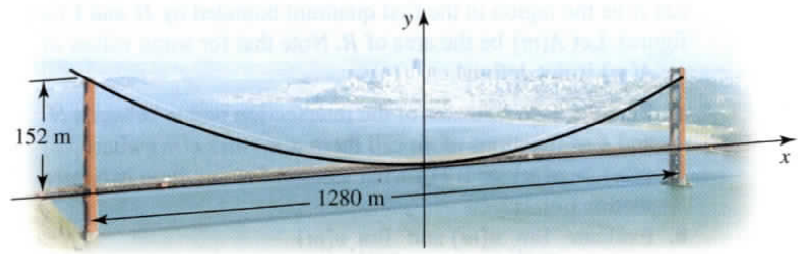×
Get Full Access to Calculus: Early Transcendentals - 1 Edition - Chapter 10.4 - Problem 84e
Get Full Access to Calculus: Early Transcendentals - 1 Edition - Chapter 10.4 - Problem 84e

×

# Solved: (See the Guided Projects for additionalISBN: 9780321570567 2

## Solution for problem 84E Chapter 10.4

Calculus: Early Transcendentals | 1st Edition

• Textbook Solutions
• 2901 Step-by-step solutions solved by professors and subject experts
• Get 24/7 help from StudySoup virtual teaching assistantsCalculus: Early Transcendentals | 1st Edition

4 5 1 353 Reviews
29
4
Problem 84E

(See the Guided Projects for additional applications of conic sections.)

Golden Gate Bridge Completed in 1937, San Francisco’s Golden Gate Bridge is 2.7 km long and weighs about 890,000 tons. The length of the span between the two central towers is 1280 m; the towers themselves extend 152 m above the roadway. The cables that support the deck of the bridge between the two towers hang in a parabola (see figure). Assuming the origin is midway between the towers on the deck of the bridge, find an equation that describes the cables. How long is a guy wire that hangs vertically from the cables to the roadway 500 m from the center of the bridge?Step-by-Step Solution:

Solution 84E

Step 1  of  2:

The given figure  is shown below ;Clearly , the given figure represents a parabola open up wards , and ( 640, 152) lies on the parabola .

We know that , the equation of a parabola open up wards with vertex at origin is :Hence , ( 640, 152) lies on the parabola=4a2694.7368

Therefore , equation of the cable is :That is ,or…………(1)

Step 2 of 2

##### ISBN: 9780321570567

The full step-by-step solution to problem: 84E from chapter: 10.4 was answered by , our top Calculus solution expert on 03/03/17, 03:45PM. Since the solution to 84E from 10.4 chapter was answered, more than 727 students have viewed the full step-by-step answer. The answer to “?(See the Guided Projects for additional applications of conic sections.)Golden Gate Bridge Completed in 1937, San Francisco’s Golden Gate Bridge is 2.7 km long and weighs about 890,000 tons. The length of the span between the two central towers is 1280 m; the towers themselves extend 152 m above the roadway. The cables that support the deck of the bridge between the two towers hang in a parabola (see figure). Assuming the origin is midway between the towers on the deck of the bridge, find an equation that describes the cables. How long is a guy wire that hangs vertically from the cables to the roadway 500 m from the center of the bridge?” is broken down into a number of easy to follow steps, and 114 words. Calculus: Early Transcendentals was written by and is associated to the ISBN: 9780321570567. This textbook survival guide was created for the textbook: Calculus: Early Transcendentals, edition: 1. This full solution covers the following key subjects: bridge, towers, cables, roadway, see. This expansive textbook survival guide covers 112 chapters, and 5248 solutions.

Unlock Textbook Solution